Home > ACC7 > Chapter cc29 > Lesson cc29.1.2 > Problem9-34

9-34.
1. For each diagram below, identify each set of angles as complementary, supplementary, or vertical. Then use what you know about the properties of angles to find the measure of each angle labeled with a variable. Homework Help ✎

1.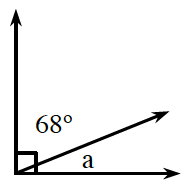2.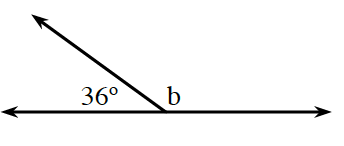3.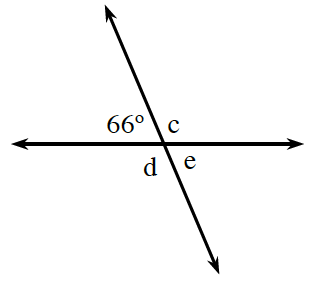4.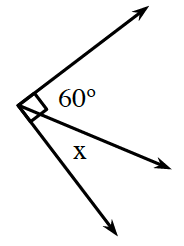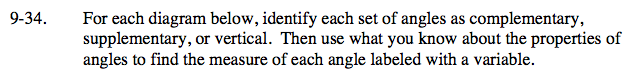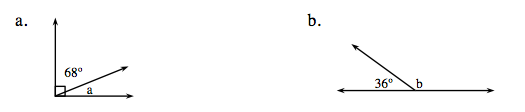Write an equation relating 68° and a.
68° + a = 90°

Subtract 68° from both sides.
a = 90° − 68° = 22°

22°; they are complementary angles

These angles are supplementary, so 36° + b = 180°. What is the measure of b?e and 66° are vertical angles, so they are equal in measure. d and c are also vertical angles.

Angle c is 114°. Use this to help you find d and e.

Use part (a) to help you solve this problem.